11月销量再创新高 哈弗神车热销势头进无止尽

在刚刚过去的11月，哈弗品牌销量再创新高，H&F双系齐发力突破10万辆大关，达到106,390辆，环比暴增25.23%，继续保持大幅上涨。这已经是哈弗品牌自今年9月以来连续第三个月环比大幅增长，增长势头锐不可当，成为2018年车市最耀眼的存在。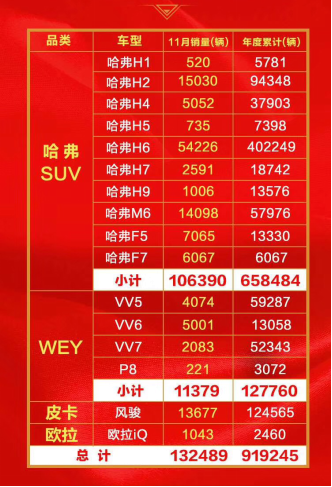在中国车市整体增速放缓的大环境下，哈弗SUV一路呈现逆势高涨的发展态势，不仅彰显了哈弗作为“中国SUV领导者”的强大实力，也为中国汽车市场的发展注入了一剂“强心剂”，树立了自主品牌不断向上突破的典范。在如此强劲势头下，哈弗品牌也即将迎来全球500万销量的达成,再一次创造车坛奇迹。这不仅仅是哈弗SUV的骄傲，更是 “中国制造”的骄傲！

那么，在辉煌荣耀的背后，哈弗SUV逆势持续热销的秘诀究竟是什么呢？

秘诀一：以精准营销抢先制胜市场 哈弗喜迎全球500万

近年来，随着SUV市场的爆发，更多的车企加入进来，SUV市场已结束“蓝海模式”进入到“红海期”，竞争愈发激烈。面对新一轮的激烈竞争，哈弗始终坚持以市场和用户需求为导向，用敏锐的目光洞察市场的变化，并做出快速响应。

为此，面对中国汽车市场增速放缓态势，哈弗主动出击，自9月开始，哈弗抢先发动攻势，推出一系列重磅促销政策，其中开展的“纯自主迎贸易战，哈弗神车降两万”促销活动迅速激发了市场活力，助力哈弗SUV销量逆市高扬，再现一车难求局面，使得哈弗成为中国SUV市场绝对的王者。

进入12月，哈弗再度出手，推出“喜迎全球500万，哈弗神车至高综合优惠3.2万，新车享50%购置税补贴”的终端钜惠活动。该项活动更是被称为车市年度之最，在行业内中实属首创，率先打响了2018年车市收官大战的第一枪，为消费者带来诚意满满的购车体验，大大提振了市场信心。更值得一提的是，哈弗在宣布重磅钜惠的同时，更宣布哈弗SUV作为专业SUV品牌，在全球销量即将达到500万辆。这为实现哈弗‘第一梦’，做全球SUV领导者提供了强大助力。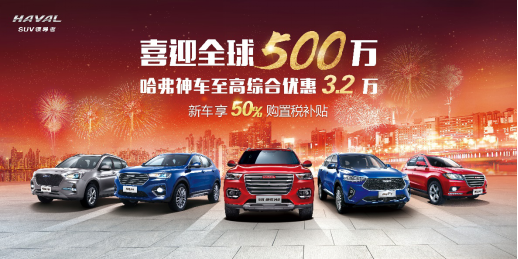同时，面对消费群体的年轻化趋势，哈弗品牌全面发力年轻化营销。2018年，哈弗全力赞助了湖南卫视跨年演唱会，朝着年轻人喜爱的娱乐营销迈出了重要一步；同时，哈弗也成为了2018年世界杯转播顶级合作伙伴，通过打造体育营销大事件为品牌传播再添重磅砝码；近期哈弗又与《蒙面唱将3》结缘，不仅成为该栏目的冠名商，还对节目进行了深度植入。可以说，这些营销举措不仅更贴合消费者的喜好，而且也进一步加强了哈弗与消费者的情感沟通与互动，起到了深得人心的效果。由此可以看到，哈弗品牌的营销战略已渐入佳境，在赢得市场与消费者高度认可的同时，也将助推品牌影响力更上新台阶。

秘诀二：实现H&F双系布局全覆盖，夯实市场统治地位

面对消费需求多样化以及快速升级趋势，哈弗SUV精准的产品布局和强大的产品力也是哈弗神车热销的一大助力。在消费升级的大环境下，为了充分满足不同消费者的消费需求，2018年，哈弗在继续丰富和优化H系的同时，发布了哈弗全新系列F系。H&F系双系合璧，全面覆盖各细分市场。其中，H系打造的是中国最好用的家用SUV，以高性价比、高实用性打动用户，成为国民心目中的爆款“神车”，而全新F系则更前卫、更时尚、更动感，通过创新设计与智能科技满足年轻用户更多个性化的需求。

更值得一提的是，在哈弗刚刚公布的11月销量数据中，两大核心主力H&F系表现出众，进一步夯实了哈弗SUV的绝对市场统治地位。而在H&F双系的助力下，可以预见，12月哈弗SUV将保持持续热销势头，领衔群雄。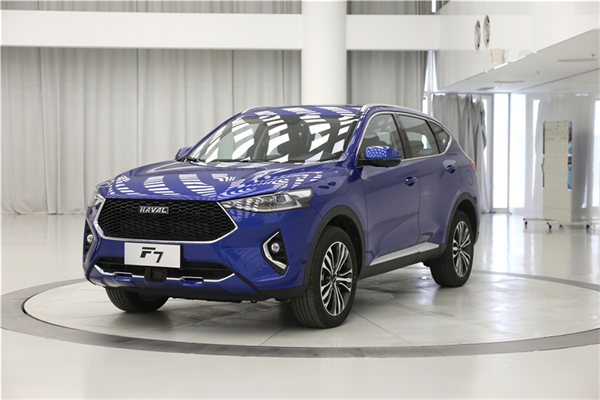秘决三：以科技创新为第一生产力 开启哈弗“智联新时代”

在加强产品布局的同时，哈弗品牌始终不忘初心，将技术创新作为品牌的第一生产力。在敏锐洞察到智能化是汽车市场未来的发展方向时，哈弗品牌毅然扛起了智能化的大旗，将大力发展智能技术，打造高品质、高性价比移动智能终端作为品牌的核心战略之一。对于智能互联技术，哈弗品牌以基础技术、用户思维、个性化服务，三位一体构建全场景化智能服务体系；同时，哈弗与百度等多家互联网行业巨头进行合作开发，并取得了丰收成果。

目前，哈弗F系产品和H系产品均已拥有 4G 智能互联系统，未来，哈弗品牌旗下产品将全面应用小度OS系统。哈弗品牌全面进入“智联新时代”。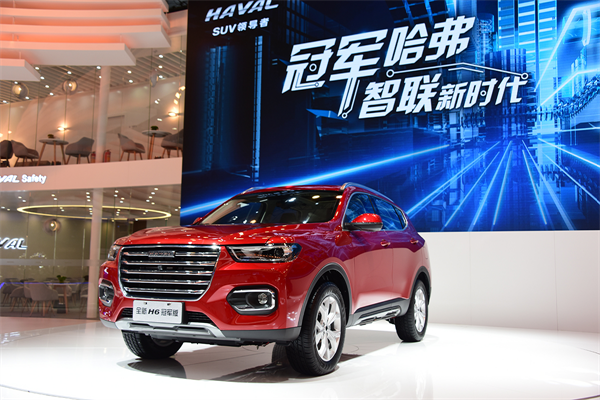此外，在动力、安全等核心技术方面，哈弗品牌同样拥有领先优势。其中哈弗旗下车型搭载的“双十佳”动力总成系统，完全由长城自主研发而成，其中1.5GDIT发动机，曾荣获“中国心”十佳发动机称号，7DCT湿式双离合变速器则被誉为“世界十佳变速器”。这套黄金动力组合成功打破了多项国际专利壁垒，助力哈弗在技术上实现质的飞越。

与此同时，哈弗一直致力于打造“最安全的SUV”，尤其在智能安全方面，ACC自适应巡航、半自动泊车、360°环视等主动安全配置不断应用在主销车型上，为用户提供了更全面的驾乘安全。

毫无疑问，哈弗在技术上取得的丰厚成果，始终离不开其一直坚持的“过度投入”战略以及自主创新精神。这也为哈弗品牌铸就了发展之魂，使其无惧挑战，勇往直前，锐意进取，引领风潮。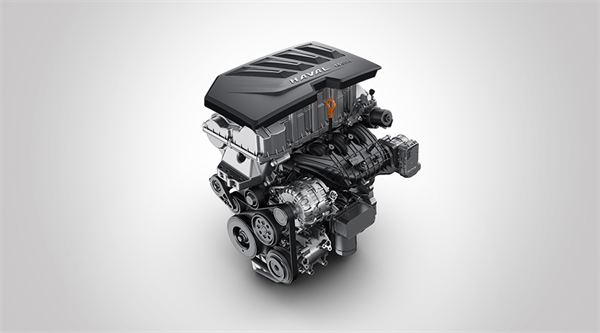综上，2018年，在市场竞争日益白热化的大环境下，哈弗作为中国SUV市场的领导者，依然能够保持SUV市场销量王者的地位，并继续领跑SUV市场。这不仅得益于其多年来厚积薄发的实力，更得益于其不断坚持自我向上突破的勇气与魄力，在营销、产品布局、技术等方面全面发力，不断提升自身综合实力，使得哈弗品牌始终保持行业领先优势，成为自主品牌的实力担当，继续引领中国汽车行业的发展。

##### 相关资讯

|分享

•已阅！握爪
•我手滑为你点赞
•128个赞！
•不明觉厉
•阅后既醉
•有钱！任性
•照片太美，我不敢看
•干货！杠杠的
•高大上
•膜拜中！
•请接受我的膝盖
•猫哥/猫妹么么哒
•神吐槽
•我只笑笑不说话
•我想静静
•窒息
##### 资讯相关车系##### 热度排行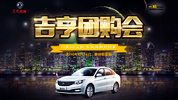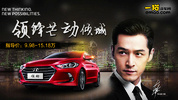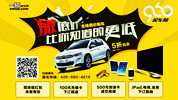• 阿斯顿·马丁
• 奥迪
• 阿尔法罗密欧
• ALPINA
• 爱驰
• AC Schnitzer

• 巴博斯
• 宝骏
• 宝马
• 保时捷
• 北汽制造
• 奔驰
• 奔腾
• 本田
• 比亚迪
• 标致
• 别克
• 宾利
• 布加迪
• 北汽威旺
• 北京
• 北汽绅宝
• 北汽幻速
• 北汽新能源
• 宝沃
• 比速汽车
• 北汽道达
• 北京汽车
• 比德文汽车
• 铂驰
• 北汽昌河

• 昌河
• 长安
• 长城
• 长安商用
• 成功汽车
• 长江EV
• 长安轻车型
• 长安欧尚
• 长安凯程
• 长安跨越
• 车驰汽车

• 大众
• 道奇
• 东风
• 东风风神
• 东风小康
• 东南
• DS
• 东风风行
• 东风风度
• 东风风光
• 电咖
• 大乘汽车
• 大运
• 东风富康

• 法拉利
• 菲亚特
• 丰田
• 福迪
• 福特
• 福田汽车
• 福汽启腾
• 风诺
• 福田

• GMC
• 广汽传祺
• 广汽吉奥
• 观致
• 国金汽车
• 广汽新能源
• 广汽集团
• 国机智骏

• 哈飞
• 海格
• 海马
• 华泰
• 黄海
• 恒天
• 红旗
• 哈弗
• 华颂
• 华凯
• 华泰新能源
• 汉腾汽车
• 华利
• 红星汽车
• HYCAN合创
• 汉龙汽车

• Jeep
• 江淮
• 江铃
• 捷豹
• 金杯
• 九龙
• 吉利汽车
• 金旅
• 金龙
• 江铃集团轻汽
• 江铃集团新能源
• 君马汽车
• 捷途
• 钧天
• 捷达
• 几何汽车

• 开瑞
• 凯迪拉克
• 科尼赛克
• 克莱斯勒
• KTM
• 卡威
• 凯翼
• 康迪
• 康迪电动汽车集团
• 开沃汽车
• 卡升

• 猎豹汽车
• 兰博基尼
• 劳斯莱斯
• 雷克萨斯
• 雷诺
• 理念
• 力帆
• 莲花汽车
• 林肯
• 铃木
• 陆风
• 路虎
• 路特斯
• 领志
• 领克
• 零跑汽车
• Lorinser
• LOCAL MOTORS
• LITE
• 领途汽车
• 理想汽车
• 罗夫哈特
• 力帆汽车

• MG
• MINI
• 马自达
• 玛莎拉蒂
• 迈凯伦
• 摩根
• 名爵
• 迈莎锐
• 迈迈

• 纳智捷
• 哪吒汽车

• 讴歌
• 欧宝
• 欧朗
• 欧拉
• 欧尚汽车

• 帕加尼
• Polestar极星

• 奇瑞
• 启辰
• 起亚
• 前途
• 庆铃汽车
• 骐铃汽车
• 全球鹰
• 乔治·巴顿

• 日产
• 荣威
• 瑞麒汽车
• 如虎
• 瑞驰
• 瑞麒
• 瑞驰新能源
• 容大智造

• 上汽大通
• smart
• 三菱
• 双环
• 双龙
• 斯巴鲁
• 斯柯达
• 萨博
• 思铭
• 赛麟
• SWM斯威汽车
• 上汽MAXUS
• SRM鑫源
• 思皓
• SHELBY
• 上喆

• TESLA
• 泰卡特
• 腾势
• 特斯拉
• 天际汽车

• 威麟
• 威兹曼
• 沃尔沃
• 五菱汽车
• 五十铃
• 潍柴英致
• WEY
• 蔚来
• 威马汽车
• 潍柴汽车

• 现代
• 雪佛兰
• 雪铁龙
• 西雅特
• 新特汽车
• 小鹏汽车
• 新宝骏

• 野马汽车
• 一汽
• 依维柯
• 英菲尼迪
• 永源
• 驭胜
• 云度
• 宇通客车
• 云雀汽车
• 远程汽车
• 一汽凌河

• 中华
• 中兴
• 众泰
• 知豆
• 之诺
• 正道汽车
• A
• B
• C
• D
• E
• F
• G
• H
• I
• J
• K
• L
• M
• N
• O
• P
• Q
• R
• S
• T
• U
• V
• W
• X
• Y
• Z

• 阿斯顿·马丁
• 奥迪
• 阿尔法罗密欧
• ALPINA
• 爱驰
• AC Schnitzer

• 巴博斯
• 宝骏
• 宝马
• 保时捷
• 北汽制造
• 奔驰
• 奔腾
• 本田
• 比亚迪
• 标致
• 别克
• 宾利
• 布加迪
• 北汽威旺
• 北京
• 北汽绅宝
• 北汽幻速
• 北汽新能源
• 宝沃
• 比速汽车
• 北汽道达
• 北京汽车
• 比德文汽车
• 铂驰
• 北汽昌河

• 昌河
• 长安
• 长城
• 长安商用
• 成功汽车
• 长江EV
• 长安轻车型
• 长安欧尚
• 长安凯程
• 长安跨越
• 车驰汽车

• 大众
• 道奇
• 东风
• 东风风神
• 东风小康
• 东南
• DS
• 东风风行
• 东风风度
• 东风风光
• 电咖
• 大乘汽车
• 大运
• 东风富康

• 法拉利
• 菲亚特
• 丰田
• 福迪
• 福特
• 福田汽车
• 福汽启腾
• 风诺
• 福田

• GMC
• 广汽传祺
• 广汽吉奥
• 观致
• 国金汽车
• 广汽新能源
• 广汽集团
• 国机智骏

• 哈飞
• 海格
• 海马
• 华泰
• 黄海
• 恒天
• 红旗
• 哈弗
• 华颂
• 华凯
• 华泰新能源
• 汉腾汽车
• 华利
• 红星汽车
• HYCAN合创
• 汉龙汽车

• Jeep
• 江淮
• 江铃
• 捷豹
• 金杯
• 九龙
• 吉利汽车
• 金旅
• 金龙
• 江铃集团轻汽
• 江铃集团新能源
• 君马汽车
• 捷途
• 钧天
• 捷达
• 几何汽车

• 开瑞
• 凯迪拉克
• 科尼赛克
• 克莱斯勒
• KTM
• 卡威
• 凯翼
• 康迪
• 康迪电动汽车集团
• 开沃汽车
• 卡升

• 猎豹汽车
• 兰博基尼
• 劳斯莱斯
• 雷克萨斯
• 雷诺
• 理念
• 力帆
• 莲花汽车
• 林肯
• 铃木
• 陆风
• 路虎
• 路特斯
• 领志
• 领克
• 零跑汽车
• Lorinser
• LOCAL MOTORS
• LITE
• 领途汽车
• 理想汽车
• 罗夫哈特
• 力帆汽车

• MG
• MINI
• 马自达
• 玛莎拉蒂
• 迈凯伦
• 摩根
• 名爵
• 迈莎锐
• 迈迈

• 纳智捷
• 哪吒汽车

• 讴歌
• 欧宝
• 欧朗
• 欧拉
• 欧尚汽车

• 帕加尼
• Polestar极星

• 奇瑞
• 启辰
• 起亚
• 前途
• 庆铃汽车
• 骐铃汽车
• 全球鹰
• 乔治·巴顿

• 日产
• 荣威
• 瑞麒汽车
• 如虎
• 瑞驰
• 瑞麒
• 瑞驰新能源
• 容大智造

• 上汽大通
• smart
• 三菱
• 双环
• 双龙
• 斯巴鲁
• 斯柯达
• 萨博
• 思铭
• 赛麟
• SWM斯威汽车
• 上汽MAXUS
• SRM鑫源
• 思皓
• SHELBY
• 上喆

• TESLA
• 泰卡特
• 腾势
• 特斯拉
• 天际汽车

• 威麟
• 威兹曼
• 沃尔沃
• 五菱汽车
• 五十铃
• 潍柴英致
• WEY
• 蔚来
• 威马汽车
• 潍柴汽车

• 现代
• 雪佛兰
• 雪铁龙
• 西雅特
• 新特汽车
• 小鹏汽车
• 新宝骏

• 野马汽车
• 一汽
• 依维柯
• 英菲尼迪
• 永源
• 驭胜
• 云度
• 宇通客车
• 云雀汽车
• 远程汽车
• 一汽凌河

• 中华
• 中兴
• 众泰
• 知豆
• 之诺
• 正道汽车
• A
• B
• C
• D
• E
• F
• G
• H
• I
• J
• K
• L
• M
• N
• O
• P
• Q
• R
• S
• T
• U
• V
• W
• X
• Y
• Z

• 阿斯顿·马丁
• 奥迪
• 阿尔法罗密欧
• ALPINA
• 爱驰
• AC Schnitzer

• 巴博斯
• 宝骏
• 宝马
• 保时捷
• 北汽制造
• 奔驰
• 奔腾
• 本田
• 比亚迪
• 标致
• 别克
• 宾利
• 布加迪
• 北汽威旺
• 北京
• 北汽绅宝
• 北汽幻速
• 北汽新能源
• 宝沃
• 比速汽车
• 北汽道达
• 北京汽车
• 比德文汽车
• 铂驰
• 北汽昌河

• 昌河
• 长安
• 长城
• 长安商用
• 成功汽车
• 长江EV
• 长安轻车型
• 长安欧尚
• 长安凯程
• 长安跨越
• 车驰汽车

• 大众
• 道奇
• 东风
• 东风风神
• 东风小康
• 东南
• DS
• 东风风行
• 东风风度
• 东风风光
• 电咖
• 大乘汽车
• 大运
• 东风富康

• 法拉利
• 菲亚特
• 丰田
• 福迪
• 福特
• 福田汽车
• 福汽启腾
• 风诺
• 福田

• GMC
• 广汽传祺
• 广汽吉奥
• 观致
• 国金汽车
• 广汽新能源
• 广汽集团
• 国机智骏

• 哈飞
• 海格
• 海马
• 华泰
• 黄海
• 恒天
• 红旗
• 哈弗
• 华颂
• 华凯
• 华泰新能源
• 汉腾汽车
• 华利
• 红星汽车
• HYCAN合创
• 汉龙汽车

• Jeep
• 江淮
• 江铃
• 捷豹
• 金杯
• 九龙
• 吉利汽车
• 金旅
• 金龙
• 江铃集团轻汽
• 江铃集团新能源
• 君马汽车
• 捷途
• 钧天
• 捷达
• 几何汽车

• 开瑞
• 凯迪拉克
• 科尼赛克
• 克莱斯勒
• KTM
• 卡威
• 凯翼
• 康迪
• 康迪电动汽车集团
• 开沃汽车
• 卡升

• 猎豹汽车
• 兰博基尼
• 劳斯莱斯
• 雷克萨斯
• 雷诺
• 理念
• 力帆
• 莲花汽车
• 林肯
• 铃木
• 陆风
• 路虎
• 路特斯
• 领志
• 领克
• 零跑汽车
• Lorinser
• LOCAL MOTORS
• LITE
• 领途汽车
• 理想汽车
• 罗夫哈特
• 力帆汽车

• MG
• MINI
• 马自达
• 玛莎拉蒂
• 迈凯伦
• 摩根
• 名爵
• 迈莎锐
• 迈迈

• 纳智捷
• 哪吒汽车

• 讴歌
• 欧宝
• 欧朗
• 欧拉
• 欧尚汽车

• 帕加尼
• Polestar极星

• 奇瑞
• 启辰
• 起亚
• 前途
• 庆铃汽车
• 骐铃汽车
• 全球鹰
• 乔治·巴顿

• 日产
• 荣威
• 瑞麒汽车
• 如虎
• 瑞驰
• 瑞麒
• 瑞驰新能源
• 容大智造

• 上汽大通
• smart
• 三菱
• 双环
• 双龙
• 斯巴鲁
• 斯柯达
• 萨博
• 思铭
• 赛麟
• SWM斯威汽车
• 上汽MAXUS
• SRM鑫源
• 思皓
• SHELBY
• 上喆

• TESLA
• 泰卡特
• 腾势
• 特斯拉
• 天际汽车

• 威麟
• 威兹曼
• 沃尔沃
• 五菱汽车
• 五十铃
• 潍柴英致
• WEY
• 蔚来
• 威马汽车
• 潍柴汽车

• 现代
• 雪佛兰
• 雪铁龙
• 西雅特
• 新特汽车
• 小鹏汽车
• 新宝骏

• 野马汽车
• 一汽
• 依维柯
• 英菲尼迪
• 永源
• 驭胜
• 云度
• 宇通客车
• 云雀汽车
• 远程汽车
• 一汽凌河

• 中华
• 中兴
• 众泰
• 知豆
• 之诺
• 正道汽车
• A
• B
• C
• D
• E
• F
• G
• H
• I
• J
• K
• L
• M
• N
• O
• P
• Q
• R
• S
• T
• U
• V
• W
• X
• Y
• Z